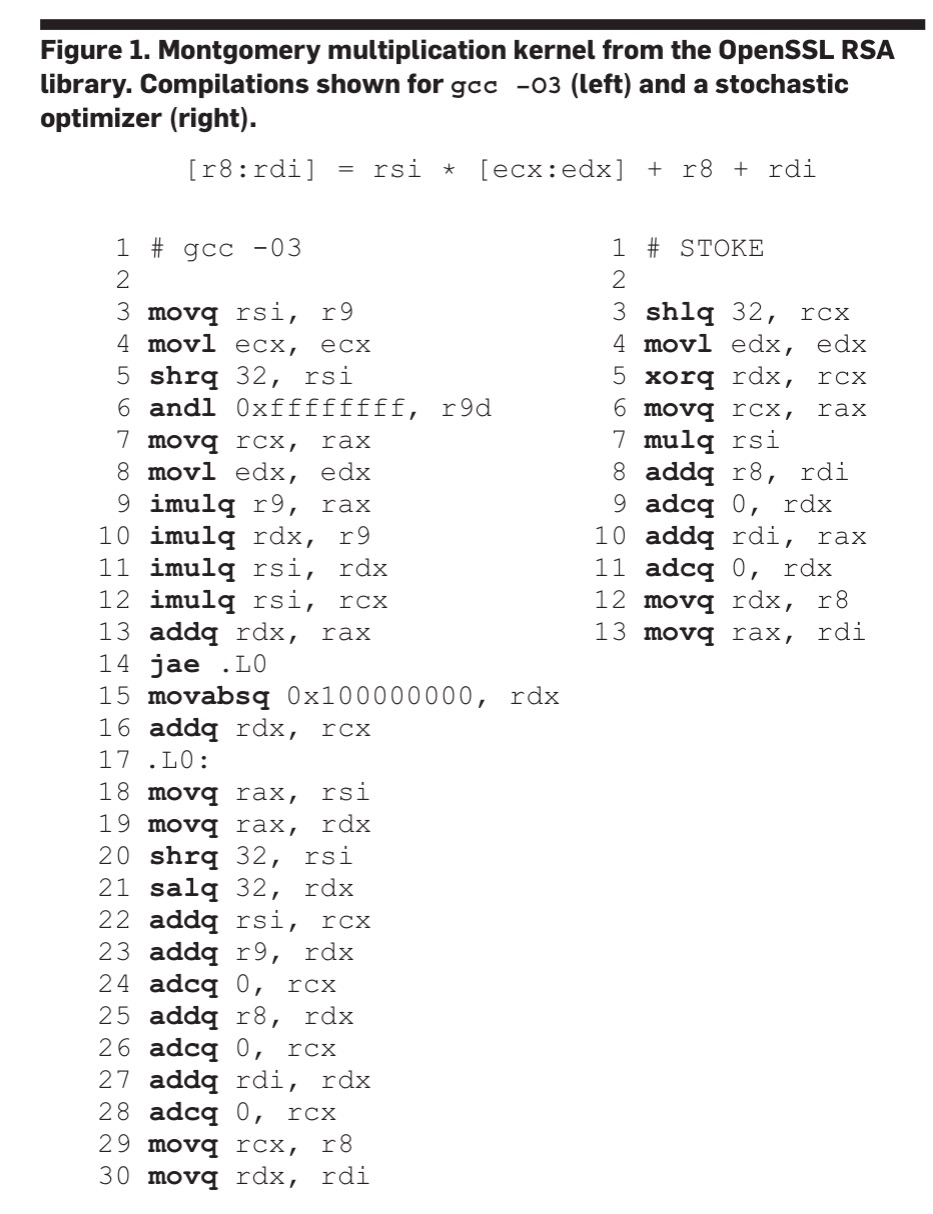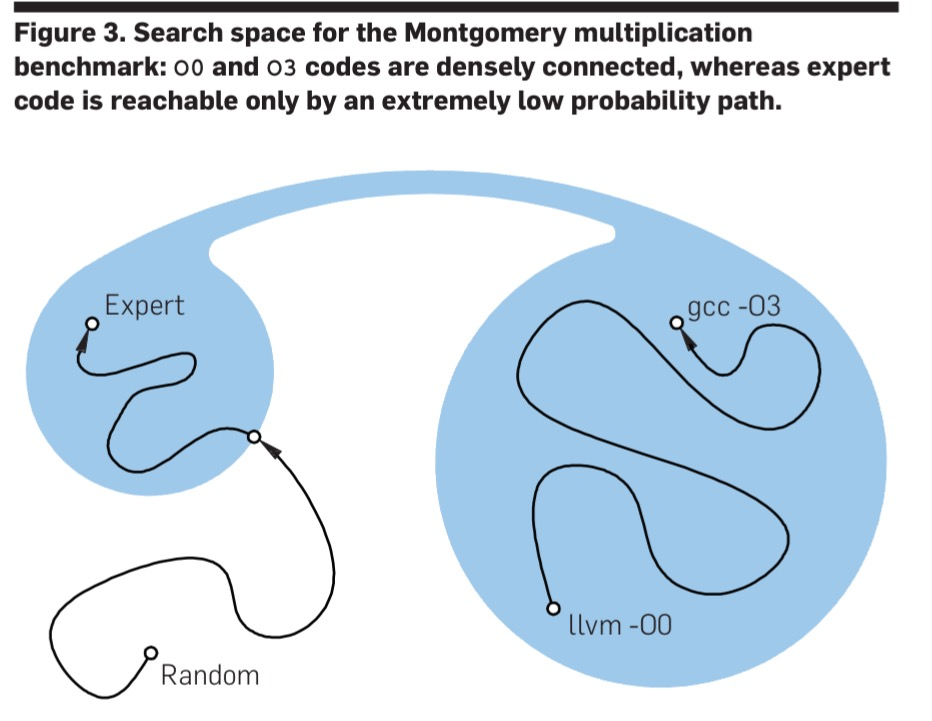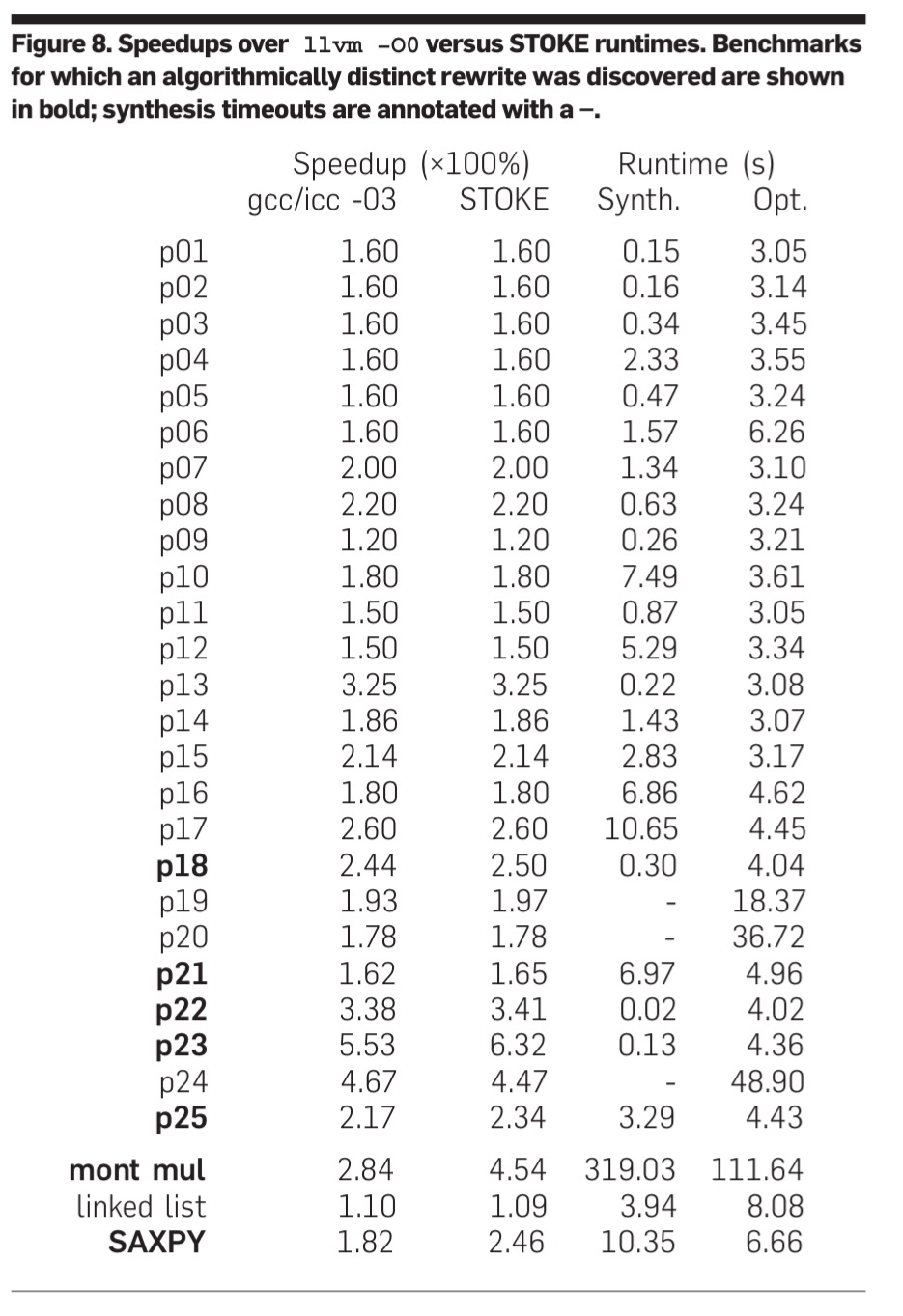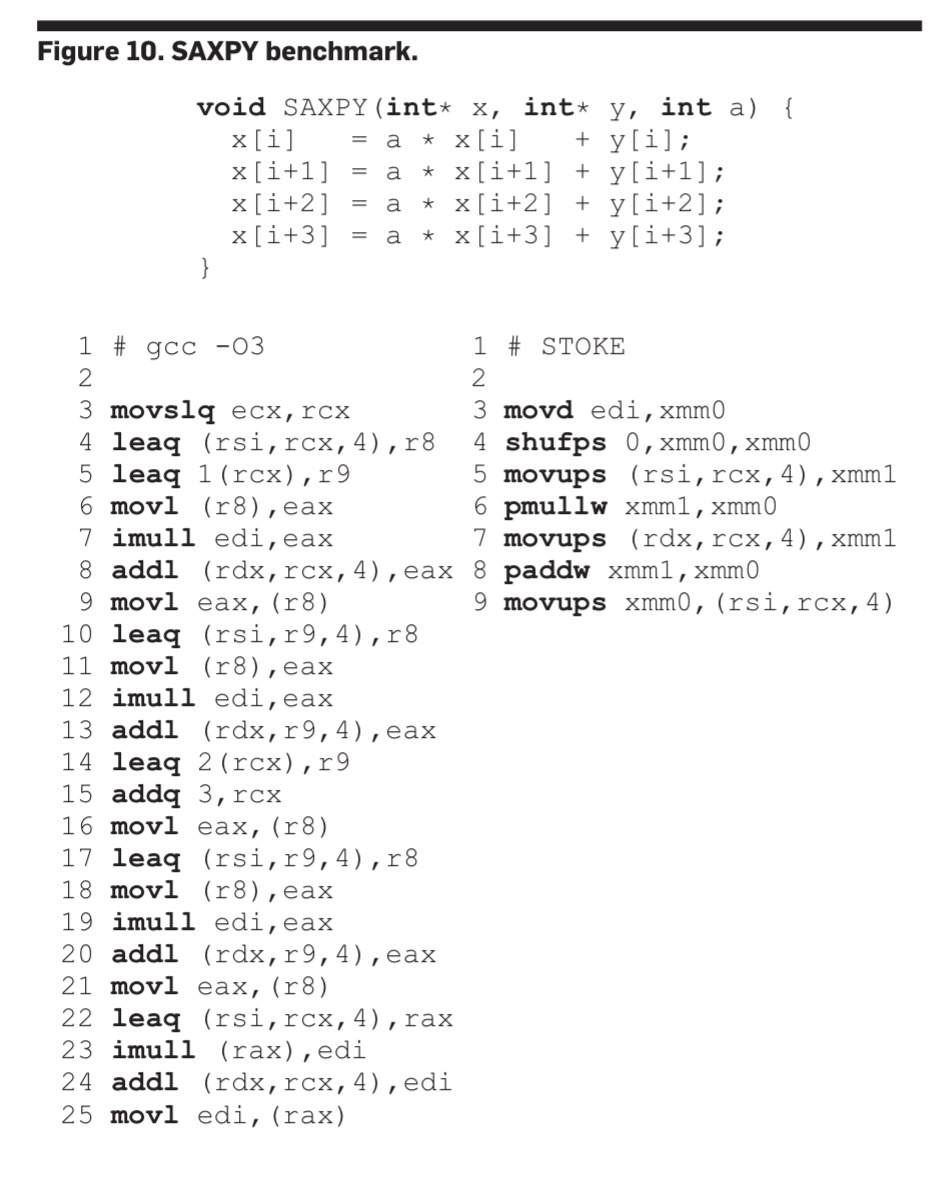# Stochastic program optimization

Stochastic program optimization Schkufza et al., CACM 2016

Yesterday we saw that DeepCoder can find solutions to simple programming problems using a guided search. DeepCoder needs a custom DSL, and a maximum program length of 5 functions. In ‘Stochastic program optimization’ Schkufza et al. also use a search strategy to generate code that meets a given specification – however, their input is a loop-free fixed-point x86_64 assembly code sequence, and the output is optimised assembler that does the same thing, but faster. There are almost 400 x86_64 opcodes, and the programs can be much longer than five instructions! The optimiser is called STOKE, and it can create provably correct sequences that match or outperform the code produced by gcc -O3 or icc -O3, and in some cases even expert handwritten assembly. (-O3 is the highest level of optimisation for gcc/icc)

Whereas a traditional optimisation phase for a compiler factors the optimisation problem into small subproblems that can be solved independently, STOKE can consider the whole program and find solutions that can only be obtained through, for example, “the simultaneous consideration of mutually dependent issues such as instruction selection, register allocation, and target-dependent optimisation.”

Here’s an illustration of STOKE at work, the output of gcc -O3 is shown on the left, and the STOKE optimised assembly on the right. In addition to being considerably shorter, it runs 1.6x faster. The original input to the optimisers (not shown) was a 116-line program produced by llvm -O0.### Optimisation approach

Because we’re not just trying to generate a correct program, but also a fast one, the problem can be framed as a cost minimisation problem with two weighted terms: one term accounting for correctness, and one for performance.

The simplest measure of correctness is functional equality. Remember that we’re given the assembly output of llvm -O0 as input, and this defines the target behaviour. So we can do black-box equality tests: consider both the target program and a candidate rewrite as functions of registers and memory contents, if they produce the same live outputs for all live inputs defined by the target then they are equal. When two programs are equal the cost of the equality term is zero (remember we’re minimising).

An optimisation is any rewrite with zero equality cost and lower performance cost (i.e., better performance) than the target.

Discovering these optimizations requires the use of a cost minimization procedure. However, in general we expect cost functions of this form to be highly irregular and not amenable to exact optimization techniques. The solution to this problem is to employ the common strategy of using an MCMC sampler.

Markov Chain Monte Carlo (MCMC) sampling draws elements from a probability density function such that regions of higher probability are sampled more often than regions of lower probability. When used for cost minimisation then in the limit most samples should be taken from the minimum (i.e., optimal) values of a function. The eagle-eyed reader may have spotted a small problem here – we don’t have a probability density function! But the Metropolis-Hastings algorithm will let us obtain samples from an approximate cost probability density function given that we can measure cost.

Given an overall computation budget, the algorithm maintains a current rewrite (initially equal to the target) and repeatedly draws a new sample, replacing the current rewrite with this sample if it is accepted. The acceptance criteria come from the Metropolis-Hastings algorithm: if the new sample has a lower cost (higher probability) it is always accepted, but if the new sample has a higher cost it may still be accepted, with a probability that decreases according to how much worse it is. Thus we hope to avoid the search becoming trapped in local minima.

### Application to x86_64 assembly

When we come to apply this general approach in the context of x86_64 we have to make three key decisions: (i) how to efficiently test equality, (ii) how to efficiently measure performance, and (iii) how to implement MCMC sampling.

For equality, we could use a symbolic validator, but his turns out to be too slow (less than 1000 evaluations a second on modestly sized code sequences). Instead, an approximation to full equality is used. A number of test cases are evaluated, and the cost term is actually defined based on how closely the output matches the target for each test input, based on the number of bits that differ between the outputs. This is much faster than using a theorem prover, and also gives a much smoother cost function than the stepped 1/0 (equal/unequal) symbolic equality test. Test case evaluations can be done at a rate of between 1 and 10 million per second.

Performance is also approximated, based on a static approximation of the cost of each instruction. (This is faster than compiling and then executing a program multiple times in order to eliminate transient effects).

For sampling, rewrites are represented as loop-free sequences of instructions of length l, with a special ‘unused’ token used to represent unused instruction slots. Starting with the target program, one of four possible moves is made:

• An instruction is randomly selected, and its opcode is replaced by a random opcode.
• An instruction is randomly selected, and one of its operands is replaced by a random operand.
• Two lines of code are randomly selected and interchanged.
• An instruction is randomly selected and replaced either by a random instruction or the UNUSED token. (Proposing an UNUSED token amounts to deleting an instruction, and replacing an UNUSED token is like inserting one).

The approach is also therefore a little reminiscent of evolutionary algorithms (but with a population of one!).

### STOKE

STOKE implements the above ideas. It runs a binary generated by a standard compiler under instrumentation to generate test cases (using Intel’s PinTool) for a loop-free target of interest, and then runs a set of synthesis threads. From the validated rewrites, the top 20% by performance are then re-ranked based on actual runtime (not the approximation used during generation), and the best is returned to the user.

As well as measuring the actual runtime of the top results, we also want to know that the rewrite is truly equivalent to the target:

STOKE uses a sound procedure to validate the equality of loop-free code sequences. Both target and rewrite are converted into SMT formulae in the quantifier free theory of bit-vector arithmetic used by Z3, producing a query that asks whether both sequences produce the same side effects on live outputs when executed from the same initial machine state. Depending on type, registers are modeled as between 8- and 256-bit vectors, and memory is modeled as two vectors: a 64-bit address and an 8-bit value (x86_64 is byte addressable).

STOKE can generate code that lies in an entirely different region of the search space to the original target code (and it differs from standard optimisers in this respect). This enables it to generate expert-level solutions, but in the original formulation it only does so with low probability.Dividing the cost minimisation process into two distinct phases helps to discover solutions in very different parts of the search space. In the first synthesis phase, code sequences are evaluated solely based on correctness, and then a subsequent optimization phase searches for the fastest sequence within each of the correct sequences.

### Results

STOKE was evaluated on benchmarks from the literature and from high-performance code, the results are summarised in the table below. Benchmarks p0 – p25 are from “Hacker’s Delight.”For example:The results shown in this CACM update improve on those in the original paper (3 years prior) by an order of magnitude or more.

### Synthesising controllers…

Before we close, I’d like to give a quick mention to ‘Sound and automated synthesis of digital stabilizing controllers for continuous plants,’ which looks at the generation of stable controllers for digital control systems. It turns out these are far from easy to write by hand given the challenges of time discretization, the noise introduced by A/D and D/A conversion, and the use of finite word length arithmetic. DSSynth can efficiently synthesize stable controllers for a set of intricate benchmarks taken from the literature, often in under a minute. To give you an idea of the kind of thing we’re talking about, one of the examples in the paper is a cruise control system.

… we leverage a very recent step-change in the automation and scalability of program synthesis. Program synthesis engines use a specification as the starting point, and subsequently generate a sequence of candidate programs from a given template. The candidate programs are iteratively refined to eventually satisfy the specification. Program synthesizers implementing Counter-Example Guided Inductive Synthesis (CEGIS) are now able to generate programs for highly non-trivial specifications with a very high degree of automation. Modern synthesis engines combine automated testing, genetic algorithms, and SMT-based automated reasoning.§